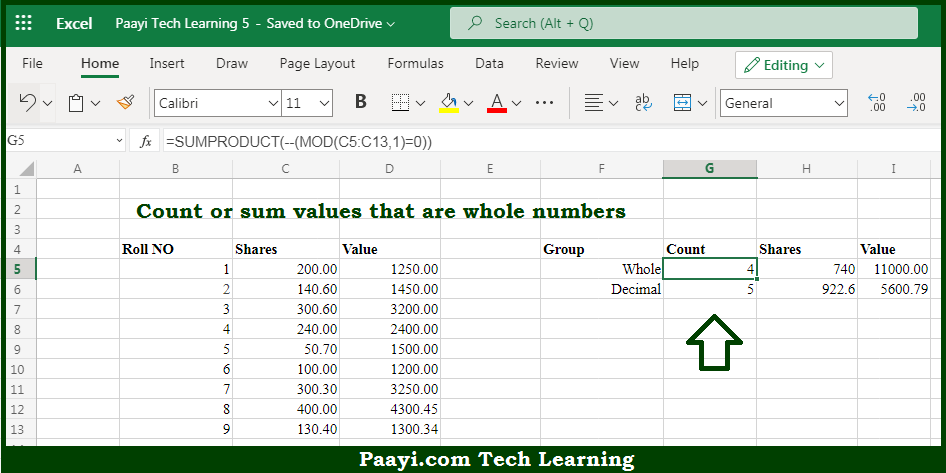# Learn How to Count or Sum Whole Numbers Only in Microsoft Excel

Written by | 0 Comments | 914 Views

In this article, you will learn how to COUNT various things in Microsoft Excel using a single or combination of functions and its purpose in Microsoft Excel. You will also get to know how to Count or Sum Whole Numbers Only and see the generic formula.

Count or Sum Whole Numbers Only in Microsoft Excel

The main purpose of this formula is to count or sum values that are whole numbers. Here we will learn how to count either count or sum the whole number values in Microsoft Excel. That implies, with the help of a formula based on the SUMPRODUCT and MOD functions you can able to count or sum the values that are whole numbers. So, with the help of this formula, you can able to how to count or sum the whole number values.

General Formula to Count or Sum Whole Numbers Only

=SUMPRODUCT(--(MOD(range,1)=0))

The Explanation for the Count or Sum Whole Numbers OnlySo we know that with the help of the given formula above you can able to count or sum values that are whole numbers. Here we will learn how to count either count or sum the whole number values in Microsoft Excel. As we know that, in the example of array provided here, TRUE values represent a whole number, and FALSE values represent a decimal number. And also that, we need to convert the TRUE and FALSE values to 1s and 0s. So, with the help of this formula, you can able to count or sum the whole numbers only.